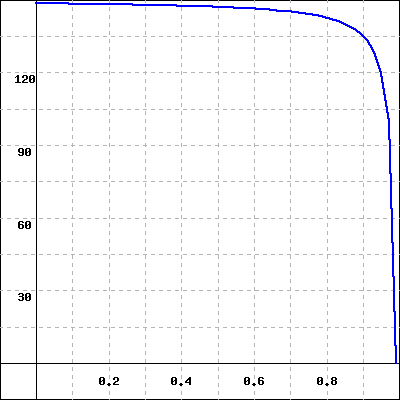The graph of a function $f(t)$ is given below.(Click on the graph for a larger version.)

Which of the following numbers could be an estimate of $\int_0^{1} f(x) dx$ accurate to two decimal places?